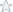MATH133 Unit 2 Individual Project A

----EQUATIONS MAY NOT BE FULLY VISIBLE: SEE PDF FILE ATTACHED TO THE RIGHT----

Typing hint: Type x2
as x^2 (shift 6 on the keyboard will give ^)
1) Solve the following quadratic equation by factoring:
a) x
2
? 6x ? 27 ? 0
Show your work here:
b) Solve the quadratic equation 3x
2
+ 2x – 16 = 0 using the quadratic formula.
Read the information in the assignment list to learn more about how to type math
symbols, such as the square root.
Show your work here:
2) Use the graph of y = x
2 + 4x - 5 to answer the following:a) Without solving the equation or factoring, determine the solution(s) to the
equation, x
2
? 4x ?5 ? 0, using only the graph.
Explain how you obtained your answer(s) by looking at the graph in a brief sentence:
b) Does this function have a maximum or a minimum?
Explain how you obtained your answer by looking at the graph in a brief sentence::
c) What are the coordinates of the vertex in (x, y) form?
d) What is the equation of the line of symmetry for this parabola?
3) The profit function for Wannamaker Trophies is P(x) = -0.4x
2
+ fx - m, where f
represents the design fee for a customer’s awards and m represents the monthly office
rent. Also, P represents the monthly profit in dollars of the small business where x is
the number of awards designed in that month.
a) If \$80 is charged for a design fee, and the monthly studio rent is \$1,600; write an
equation for the profit, P, in terms of x.
Typing hint: Type x-squared as x^2
b) How much is the profit when 50 award designs are sold in a month?
Show your work here:
c) How many award designs must be sold in order to maximize the profit? Show
your work algebraically. Trial and error is not an appropriate method of solution –
use methods taught in class.
Show your work here:d) What is the maximum profit?
Show your work here:
4) Graph the equation on the graph by completing the table and plotting the points.
You may use Excel or another web-based graphing utility.
a) y = x2
– 6x
Use the values of x provided in the table to find the y values.
x y
0
1
2
3
4
5
6
b) Place your graph here.

Complete solutions for the Math133 Individual Project A. Work is shown in detail. A++ work.

Question Files
math133_u2_ip.pdf

Other Details about the Project/Assignment
Subjects: Mathematics-> Algebra
Topic: Individual project on quadratic functions
Level: College/University
Tags:

Algebra,Math133, Unit 2 ,Indvidual,Project,solution

Price\$6.95

Math Genius
 Manny I. Member Since: Aug 2007 Customer Rating: 92.8 Projects Completed: 487 Total Earnings: Private +1 Ratings from clients: 89
Project Details
Question Files
math133_u2_ip.pdf

Subjects: Mathematics-> Algebra
Topic: Individual project on quadratic functions
Level: College/University
Tags:

Algebra,Math133, Unit 2 ,Indvidual,Project,solution

Customer Reviews
Rated 6 Times
Rating( 4.5 / 5 Stars)

Reviews of the solutions left by other users
No customer has left a detailed review for these math solutions. Be the first one to purchase them and leave a review for others!

Not exactly what you are looking for?
We regularly update our math homework solutions library and are continually in the process of adding more samples and complete homework solution sets. If you do not find what you are looking for, just go ahead and place an order for a custom created homework solution. You can hire/pay a math genius to do your homework for you exactly to your specifications.